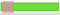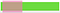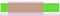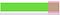Photo by Alain Wong on Unsplash

# Move Along

## Dynamic-Size Sliding Window Pattern/Technique

To solve problems like these, we could apply a brute force solution with a nested loop, but it would produce O(n²) time complexity at best. Applying the dynamic-size sliding window pattern can reduce the time complexity to O(n) and space complexity to O(1).

## Moving on

In this pattern, two pointers create a window that represents the current subsection of the data structure. One pointer is the leading edge and the other pointer is the trailing edge. This window, or chunk, is evaluated to see if it satisfies the given criteria (e.g. the sum of its values is equal to k).Sliding Window Technique — Algorithmic Mental Models

Even if the window satisfies the given criteria, the window’s size is changed to see if it can do better (e.g. create a longer/shorter subsection with the sum of its values equal to k).Sliding Window Technique — Algorithmic Mental Models

The window grows (by incrementing the leading edge) or shrinks (by incrementing the trailing edge), moving it along the data structure.Sliding Window Technique — Algorithmic Mental Models

The window keeps inching along until it reaches the stopping condition, usually the end of the data structure.Sliding Window Technique — Algorithmic Mental Models

The criteria will vary from problem to problem. Based on the problem’s criteria, the solution will usually need two variables, in addition to the pointers, to help track the result. Sometimes, an auxiliary data structure (e.g. hash table) is needed instead of a primitive data type to track a result.

## We are moving in the right direction when the problem:

• involves a data structure that is iterable (arrays, strings, etc.)
• asks to find a subsection (substring/subarray) that meets a desired condition/target value (e.g. max/min/common/longest/shortest/contains)
• does NOT have a size constraint

## Example — JavaScript

`Write a function called minSubArrayLen which accepts two parameters — an array of positive integers and a positive integer. The function should return the minimal length of a contiguous subarray of which the sum is greater than or equal to the intger passed in. If there isn’t one, return 0.Examples:minSubArrayLen([2,3,1,2,4,3], 7) // 2minSubArrayLen([2,1,6,5,4], 9) // 2minSubArrayLen([3,1,7,11,2,9,8,21,62,33,19], 52) // 1minSubArrayLen([1,4,16,22,5,7,8,9,10], 39) // 3minSubArrayLen([1,4,16,22,5,7,8,9,10], 55) // 5minSubArrayLen([4,3,3,8,1,2,3], 11) // 2minSubArrayLen([1,4,16,22,5,7,8,9,10], 95) // 0`

## Sample Problems

📚 Resources 👀

Sliding Window Technique

Sliding Window Technique — Algorithmic Mental Models

Udemy: JavaScript Algorithms and Data Structures Masterclass